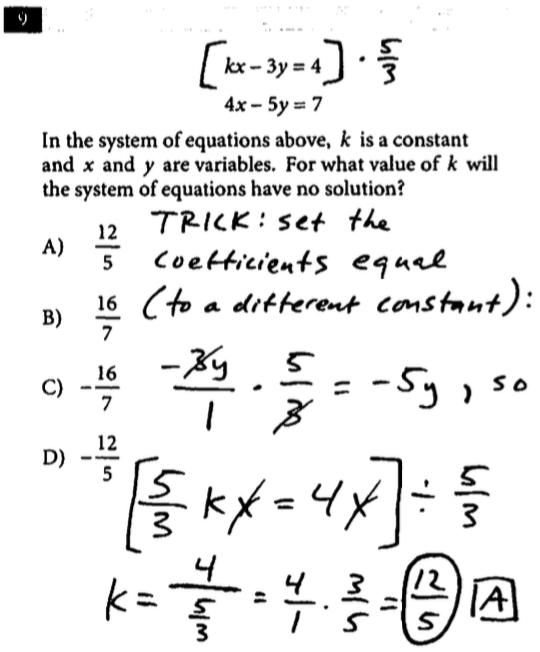# SAT Math Tips Post

## SAT Question of the Day: Test #3 Sec 3 Math NC #9

kx - 3y = 4
4x - 5y = 7

"In the system of equations above, k is a constant and x and y are variables.  For what value of k will the system of equations have no solution?"

A) 12/5
B) 16/7
C) -16/7
D) -12/5

This is a tricky one.  The concept here is that if we have a system of equations where the coefficients of the variables are the same, but they are set equal to two different constants, then the system of equations has no solution because the lines are parallel.  For example, the system of equations y = 2x +3 and y = 2x + 4 has no solution, because the lines have equal slopes but different y-intercepts.

So what I do here is multiply both sides of the equation by 5/3 to solve for k.  You will notice that the top equation is equal to 20/3, and the bottom equation is equal to 7 (close but not the same number).

The answer is Choice A, 12/5.

Attached is a visual that should help.